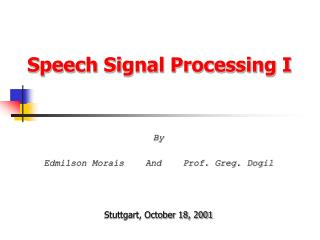DownloadDownload PresentationSpeech Signal Processing I

# Speech Signal Processing I

Télécharger la présentation## Speech Signal Processing I

- - - - - - - - - - - - - - - - - - - - - - - - - - - E N D - - - - - - - - - - - - - - - - - - - - - - - - - - -
##### Presentation Transcript

1. Speech Signal Processing I By Edmilson Morais And Prof. Greg. Dogil Stuttgart, October 18, 2001

2. Goals of the Course • Our part • Basic theoretical concepts about • Speech Signal Processing - SDSP • Waveform generation for TTS systems - TTS • Automatic Speech Recognition (Statistical approach)- ASR • Fundaments of programing in Matlab • It will be the tool used for our simulations • Your part ? • Describe and justify the important aspects and drawbacks in the algorithm. • Next term: Speech Signal Processing II • Going deeper into more Theoretical and Pratical aspects of : SSP, TTS and ASR.

3. Tutorial of Matlab • Principles of linear algebra • Vectors, Matrices, linear systems • Programing in Matlab • Variables, operators, ... • if statements, switch statements, for loops, while loops, continue statements, break statements, ... • I/O operations • Graphical visualization • Executable files • Subroutines

4. Matlab : Graphical visualization [X,Y] = meshgrid(-8:.5:8); R = sqrt(X.^2 + Y.^2) + eps; Z = sin(R)./R; mesh(X,Y,Z,'EdgeColor','black') surf(X,Y,Z,'FaceColor','red','EdgeColor','none'); camlight left; lighting phong

5. Mean squared error - E Weight Matlab : Graphical visualization – Optimization in a hiperbolic (quadratic) surface

6. SDSP : Looking through time Speech signal : Analog and digital amplitude quantization Sampling rate time

7. SDSP : Transformation and Digital filters • Transformations • Z-Transforms, Fourier transforms • Digital filters • FIR, IIR

8. SDSP – Frame based analysis Waveform multiplied for the hanning window : xw Hanning window : w Magnitude of the spectrum of xw Freq. Response of the LP-filter

9. Before smoothing Current After smoothing Current Previous Previous SDSP - Looking at frequency components through time

10. SDSP : Vector quantization Voronoi Space : Centroid and Distortion meassure

11. . O r i g i n a l S p e e c h S i g n a l C o d i n g x A – LP coeficients e – LP residue En – Prototypes Fo – Fundamental frequency U/UV – Voiced / Unvoiced transitions M a r k s Parametrization : Mapping the waveform into a set of parameters I n v e r s e F i l t e r A L P A n a l y s i s P i t c h M a r k s 1 A ( z ) A ( z ) e M a r k s P r o t o t y p e s S a m p l i n g U / U V F A E n o S t o r a g e E n v i r o m e n t D e c o d i n g F A U / U V E n o T F I R e s i d u e Reconstruction: Synthesis of the waveform from the set of parameters. P r o s o d i c Prosody : F0 Duration Amplitude S y n t h e s i s I n f o r m a t i o n S y n t h e s i s F i l t e r A ( ) z . x S y n t h e s i z e d S p e e c h S i g n a l . TTS - Waveform generation for TTS • Analysis and Resynthesis – Coding and Decoding

12. TTS - Waveform generation for TTS • Speech coding • Parametric coders, Waveform coders, Hybrid coders • TTS – Concatenative approach • Time scale and Frequency scale modifications • Spectral smoothings • Unit selection Original TTS Original Resynthesized Modified : sin(x+)

13. ASR - Automatic Speech Recognition • Front-End Signal Processing • Feature extraction • Perceptual domain, Articulatory domain • Acoustic modeling • HMM : Hidden Markov Model • ANN/HMM : Hybrid models - Artificial Neural Network and HMM • Statistical Language Modeling • N-grammars, smoothing techniques • Search : Decoding • Viterbi, Stack decoding, ...

14. ASR – HMM - Topology Ergotic model Left-right model

15. ASR – HMM – Basic principle a a a a a a a a a a a a a

16. 5 0 1 0 0 1 5 0 2 0 0 5 0 1 0 0 1 5 0 2 0 0 ( b ) ( a ) 5 0 1 0 0 1 5 0 2 0 0 5 0 1 0 0 1 5 0 2 0 0 ( c ) ( d ) ASR – HMM - Viterbi alignment

17. ASR – HMM – Forward-Backward

18. ASR – ANN/HMM

19. Evaluation : Exercises and Simulations • List of Exercises • SDSP, TTS, ASR • Simulations • SDSP • Vector quantization • TTS • Waveform Interpolation • ASR • Acoustic modeling using : HMM and ANN+HMM • Language modeling • Decoding

20. Evaluation : Report • Reports • Write the analysis and results of the simulation in a format of a paper • 4 pages, two colunms. • Sections • Abstract • Introduction • Brief theoretical description of the method • Methodology used to perform the experiment • Results • Conclusions and suggestions for further works • Bibliograph

21. Days of classes